# Frank Solutions for Class 9 Maths Chapter 3 Compound Interest

Frank Solutions for Class 9 Maths Chapter 3 Compound Interest contain solutions prepared by experienced faculty at BYJU’S. In a subject like Mathematics, it’s important for students to understand the concepts in-depth. Practising the problems on a regular basis is the main key to securing good marks in the annual examination.

Chapter 3 consists of problems in finding the amount and compound interest, according to the latest ICSE Board syllabus. The solutions are available in PDF to help students solve the exercise questions in the Frank textbook with ease. Practising these solutions on a regular basis will help them improve their problem-solving and time-management skills, which are important from the exam perspective. For more conceptual knowledge, students can download and practise Frank Solutions for Class 9 Maths Chapter 3 Compound Interest PDF from the link provided below.

## Frank Solutions for Class 9 Maths Chapter 3 Compound Interest Download PDF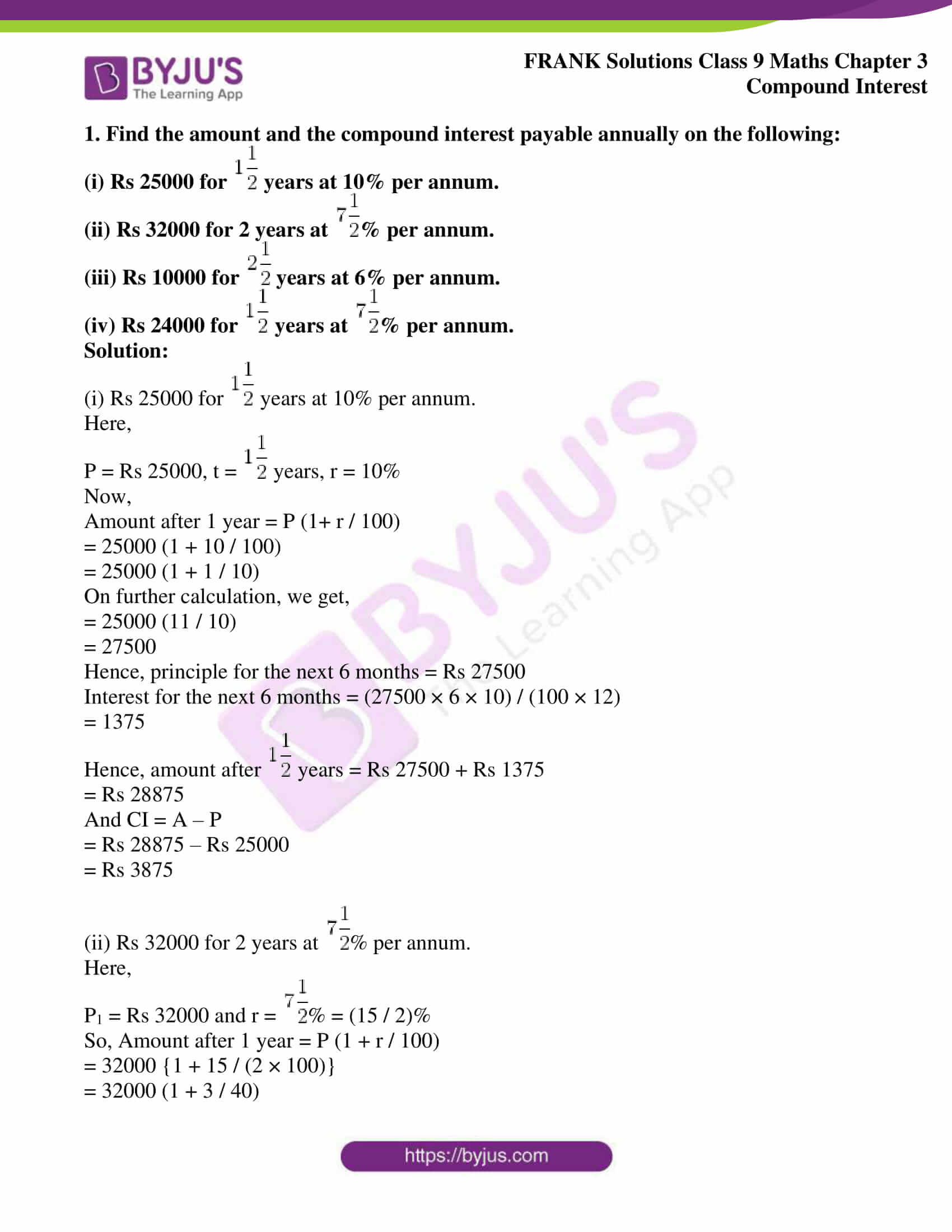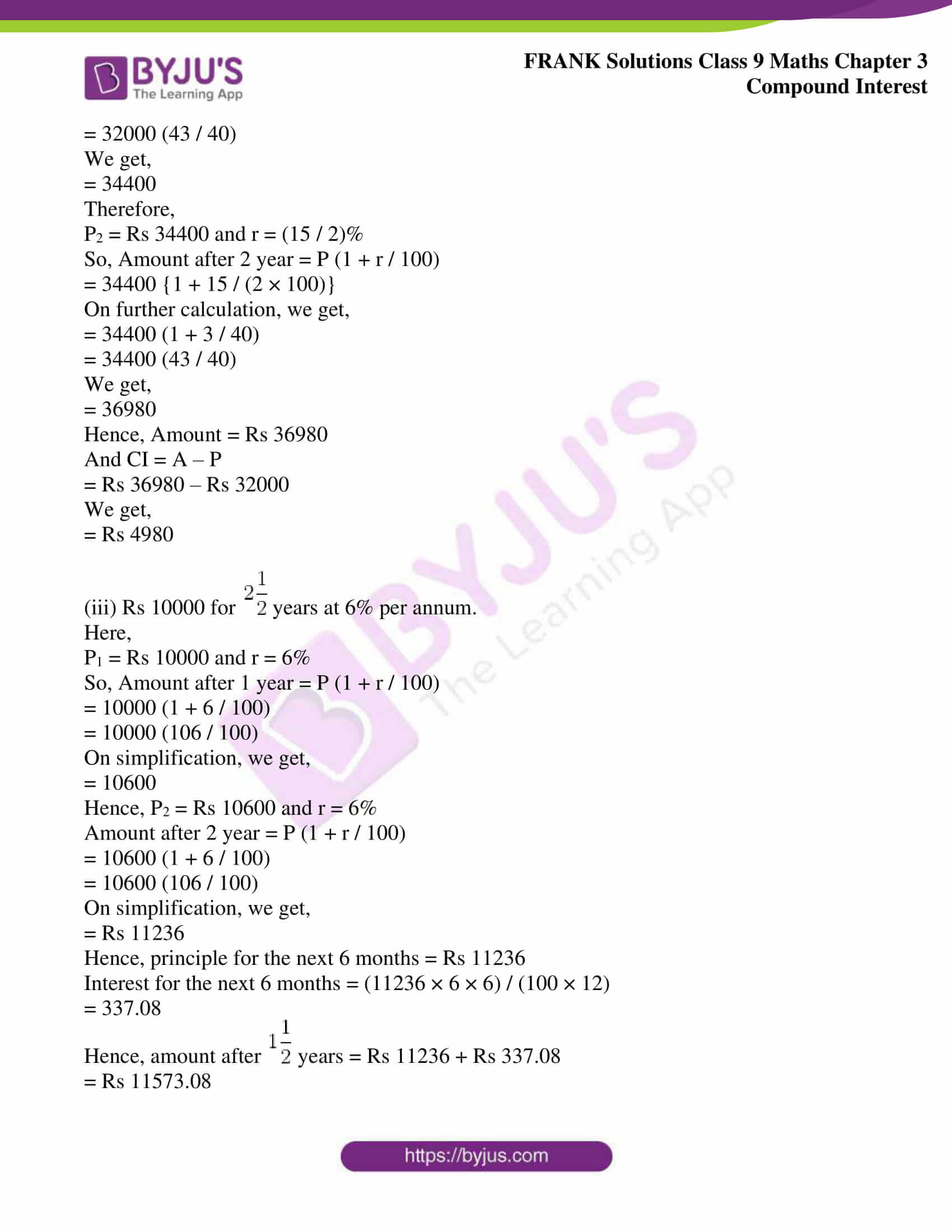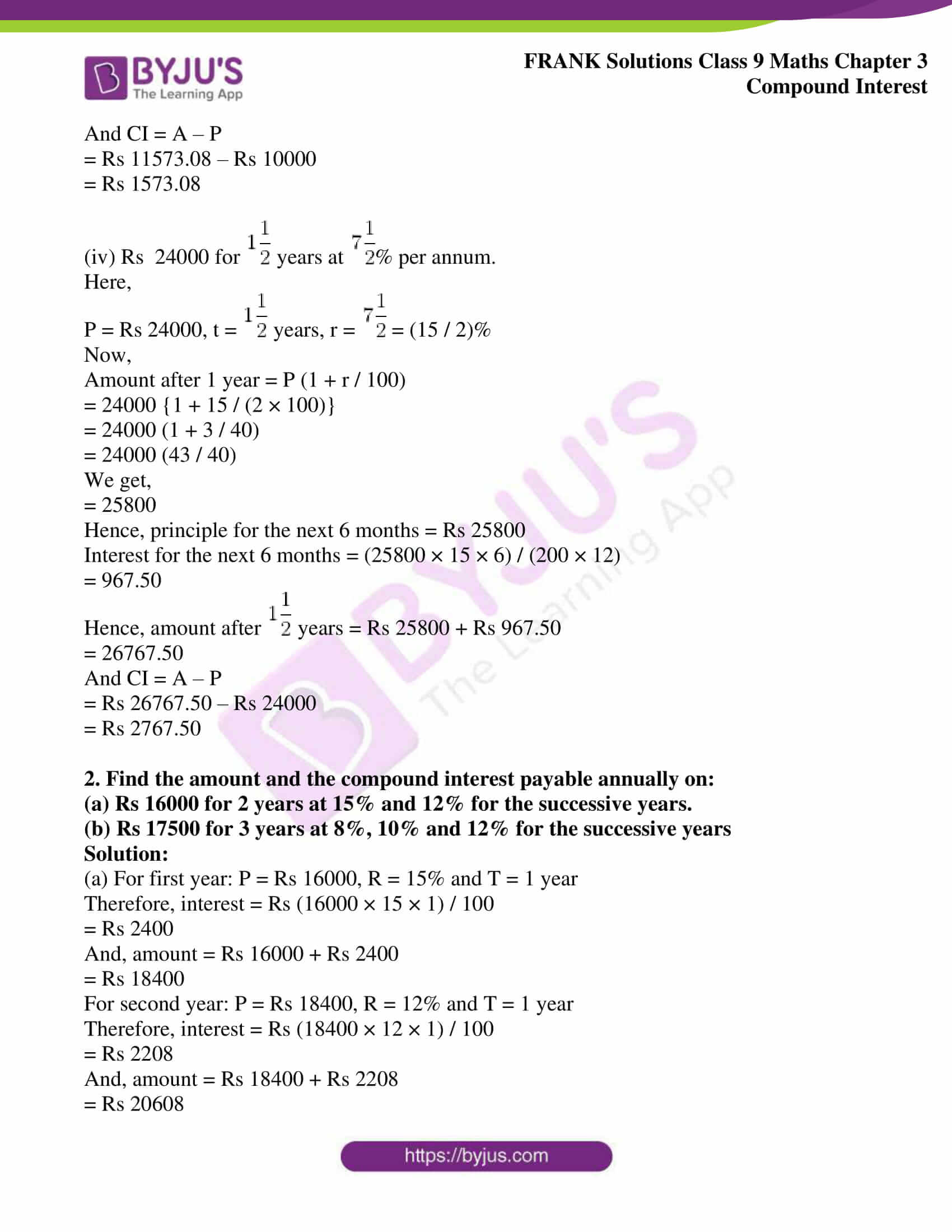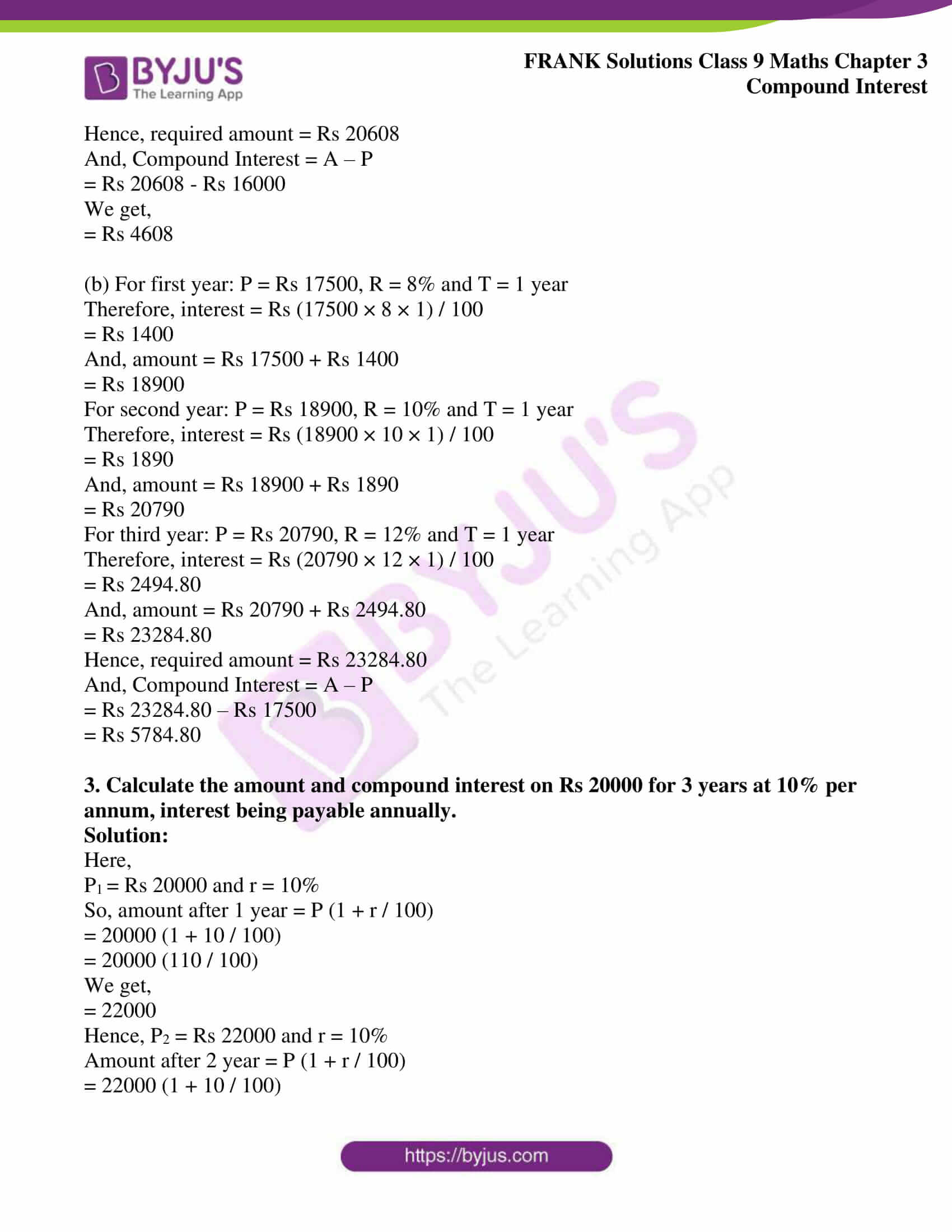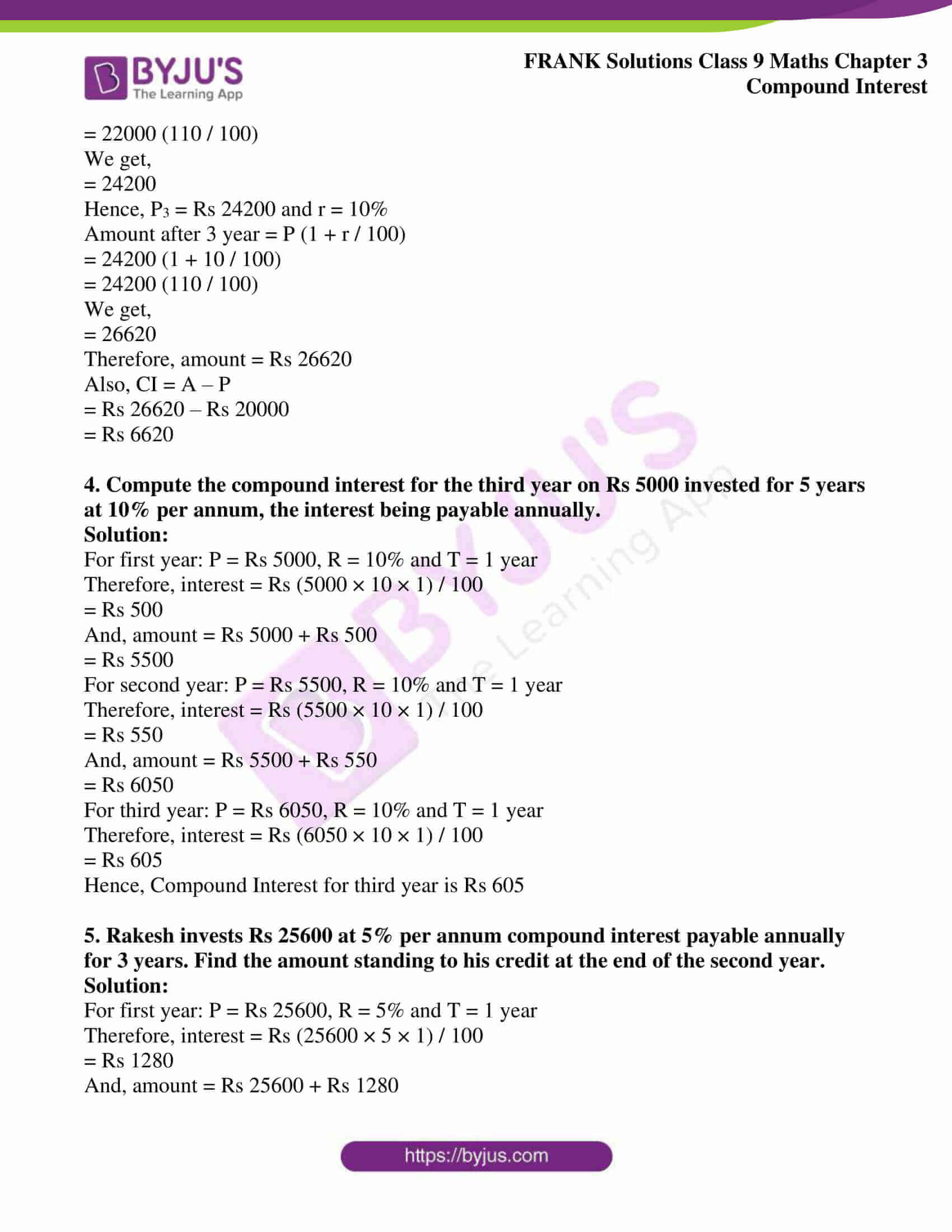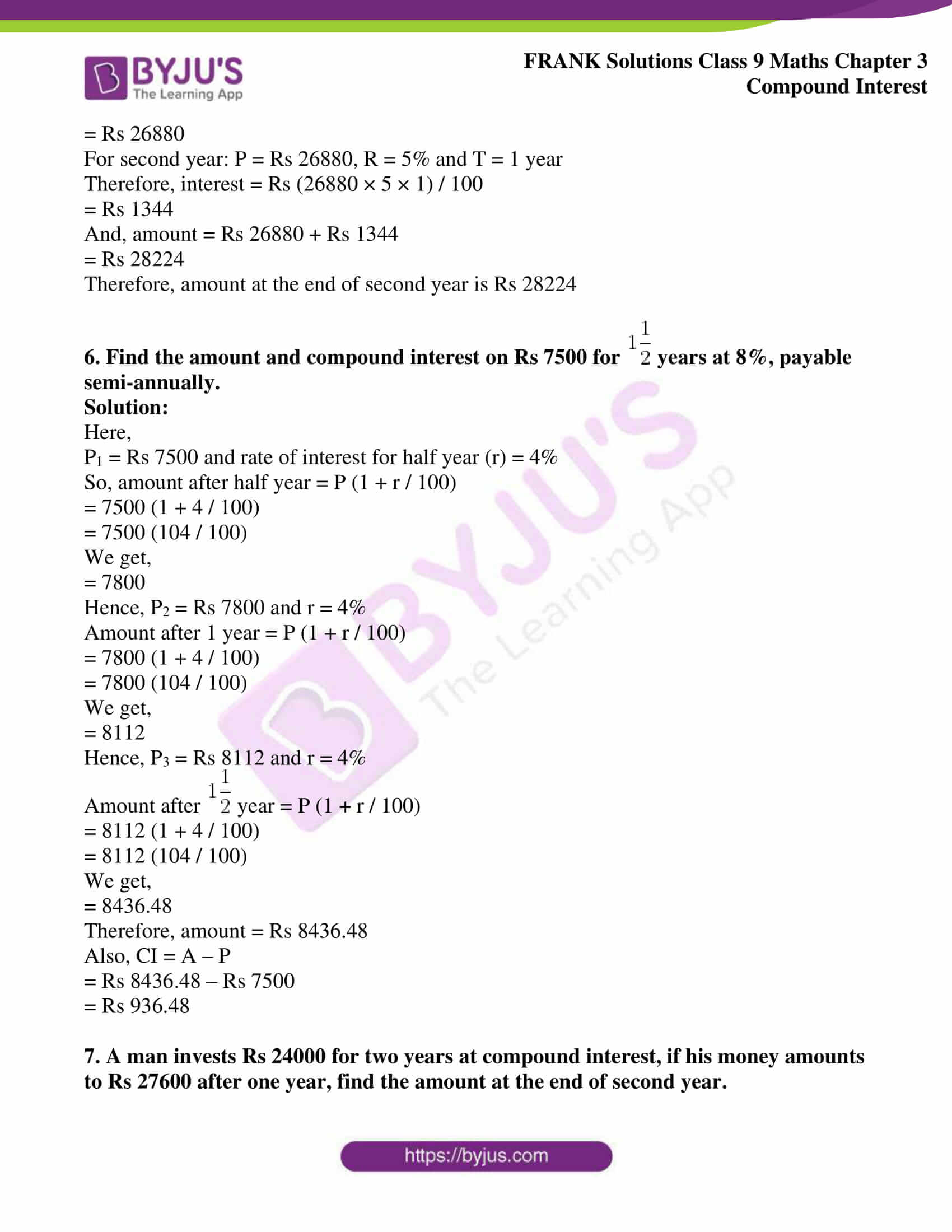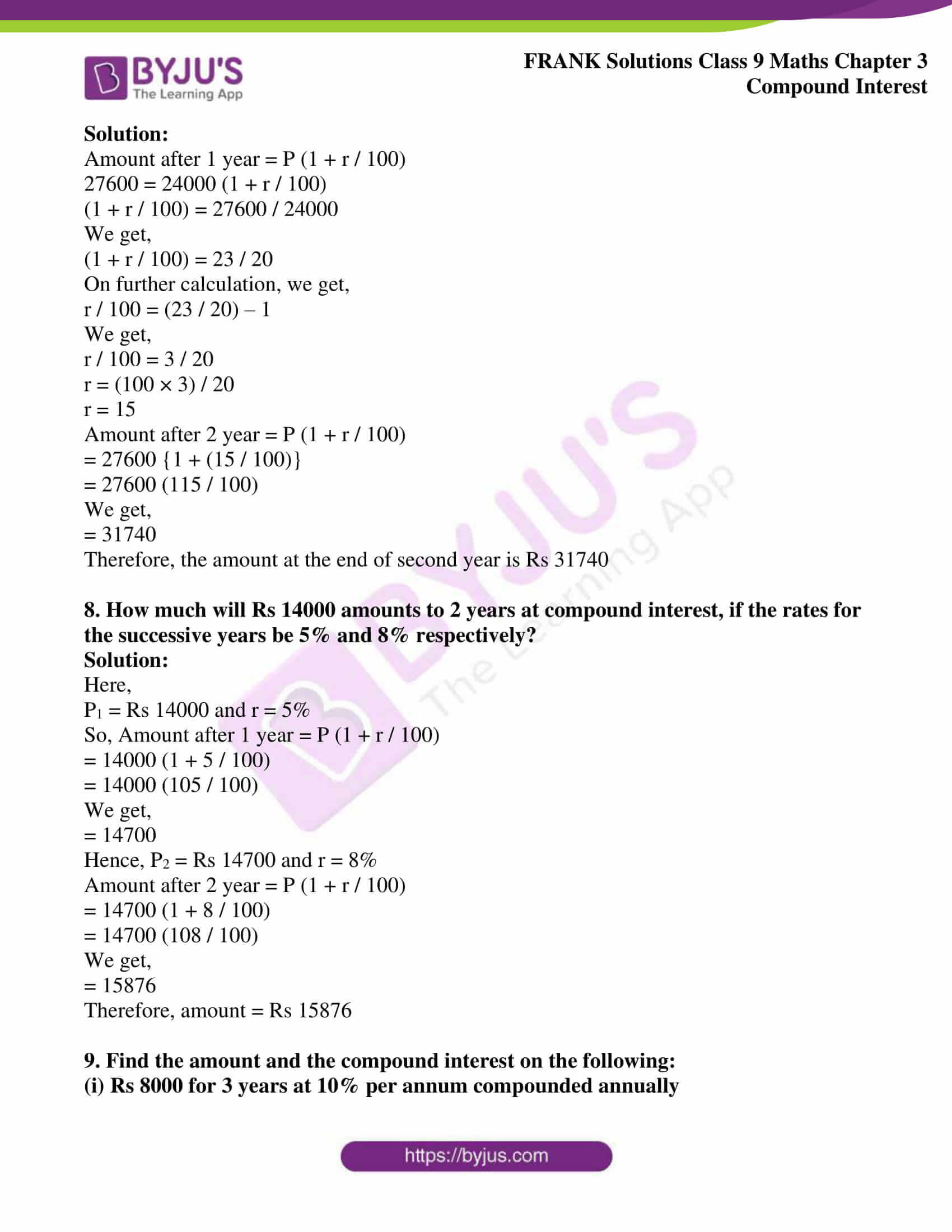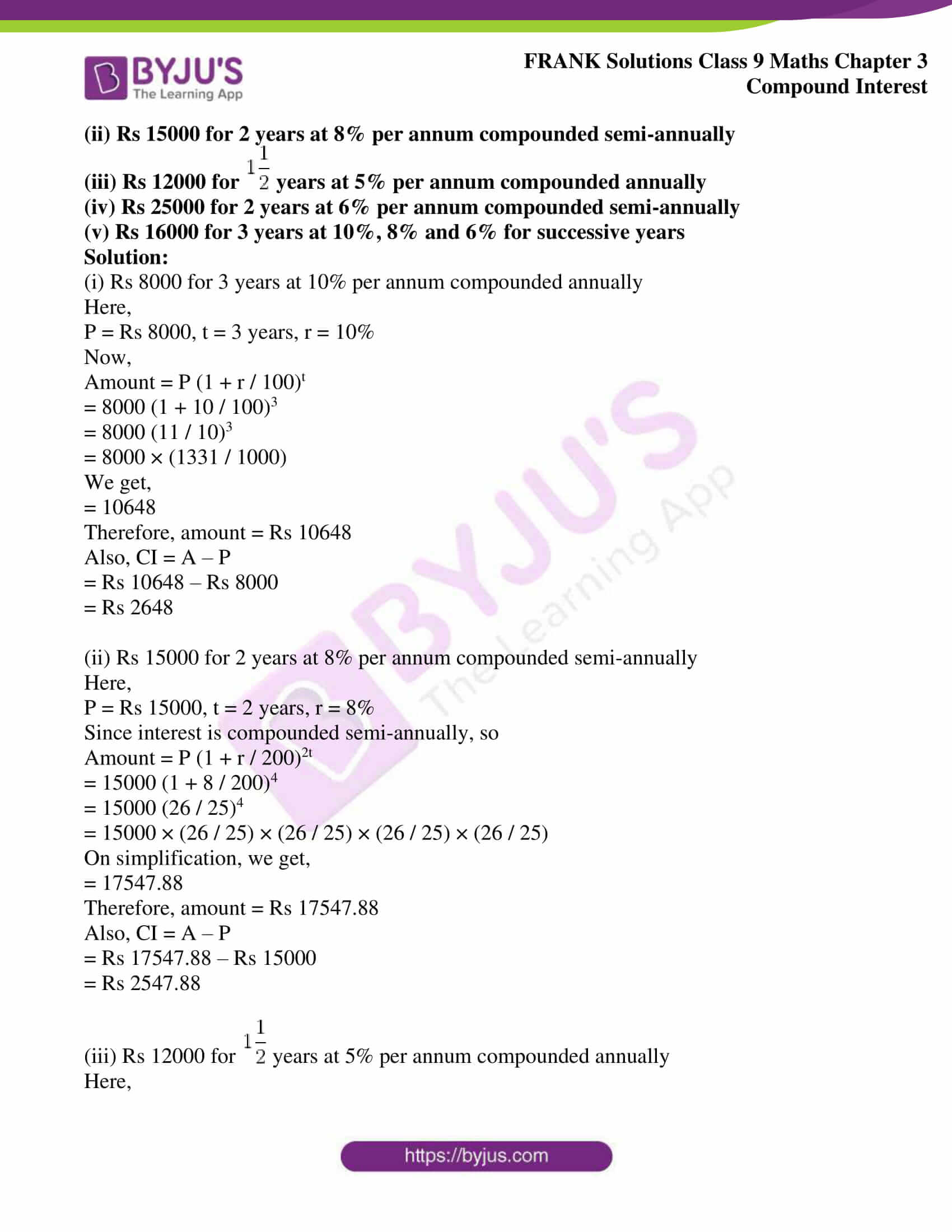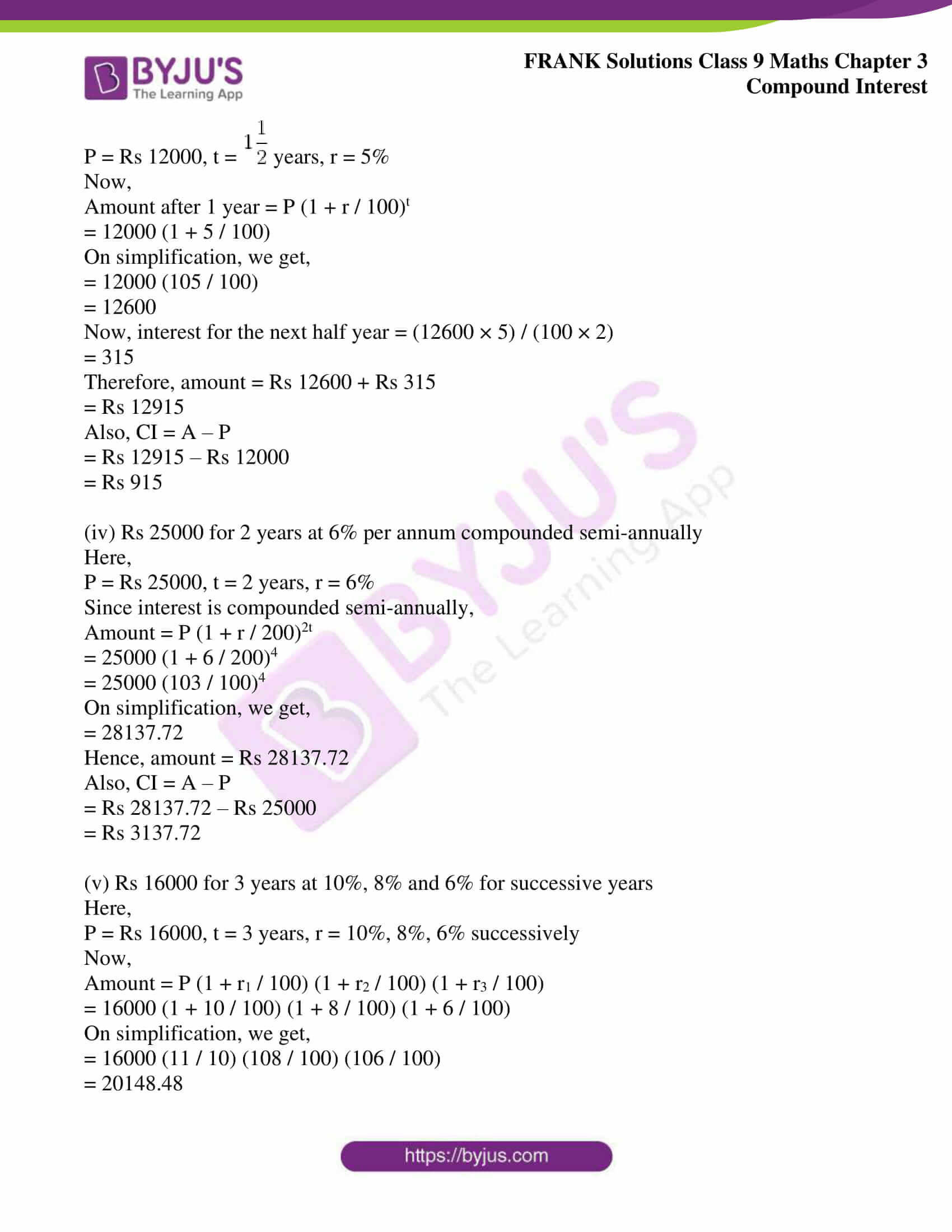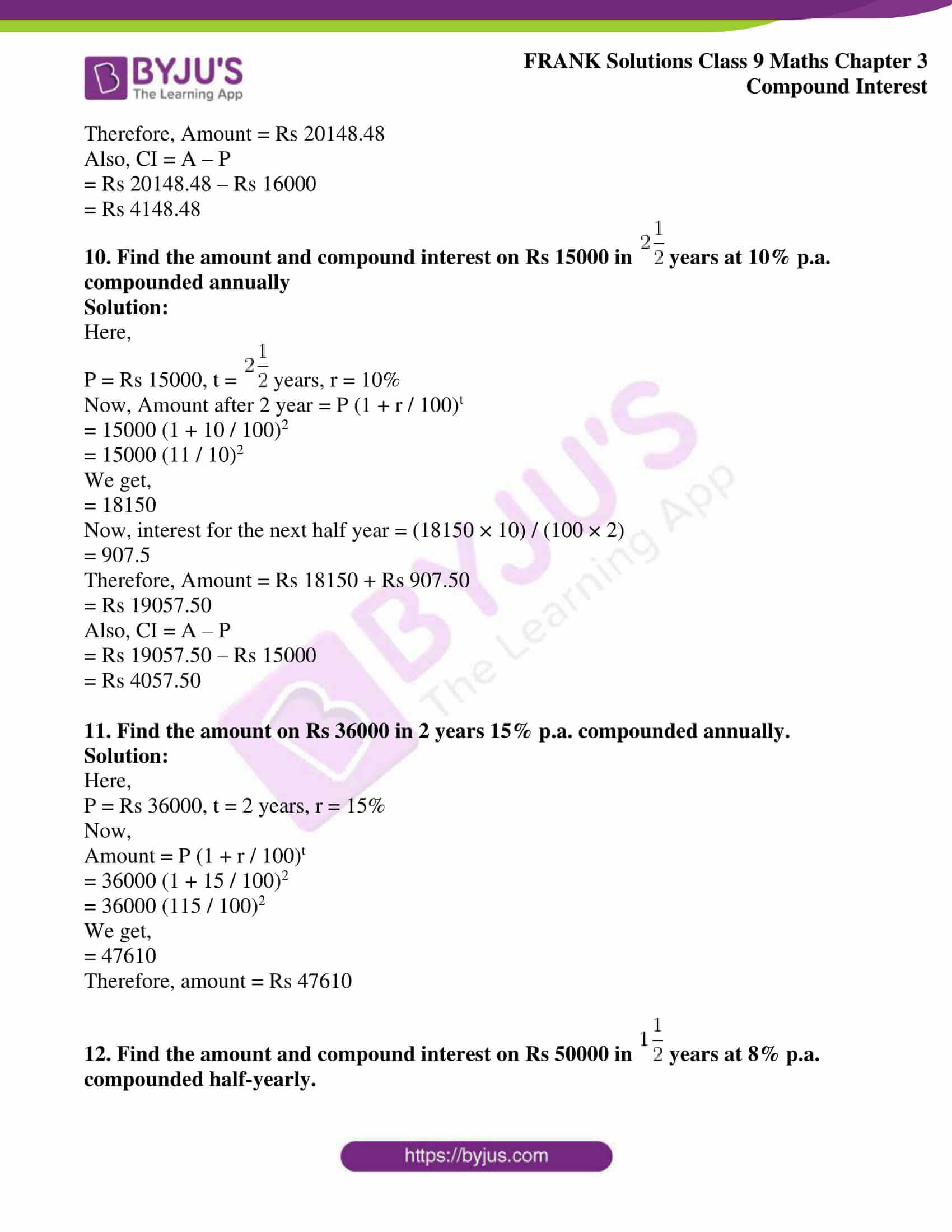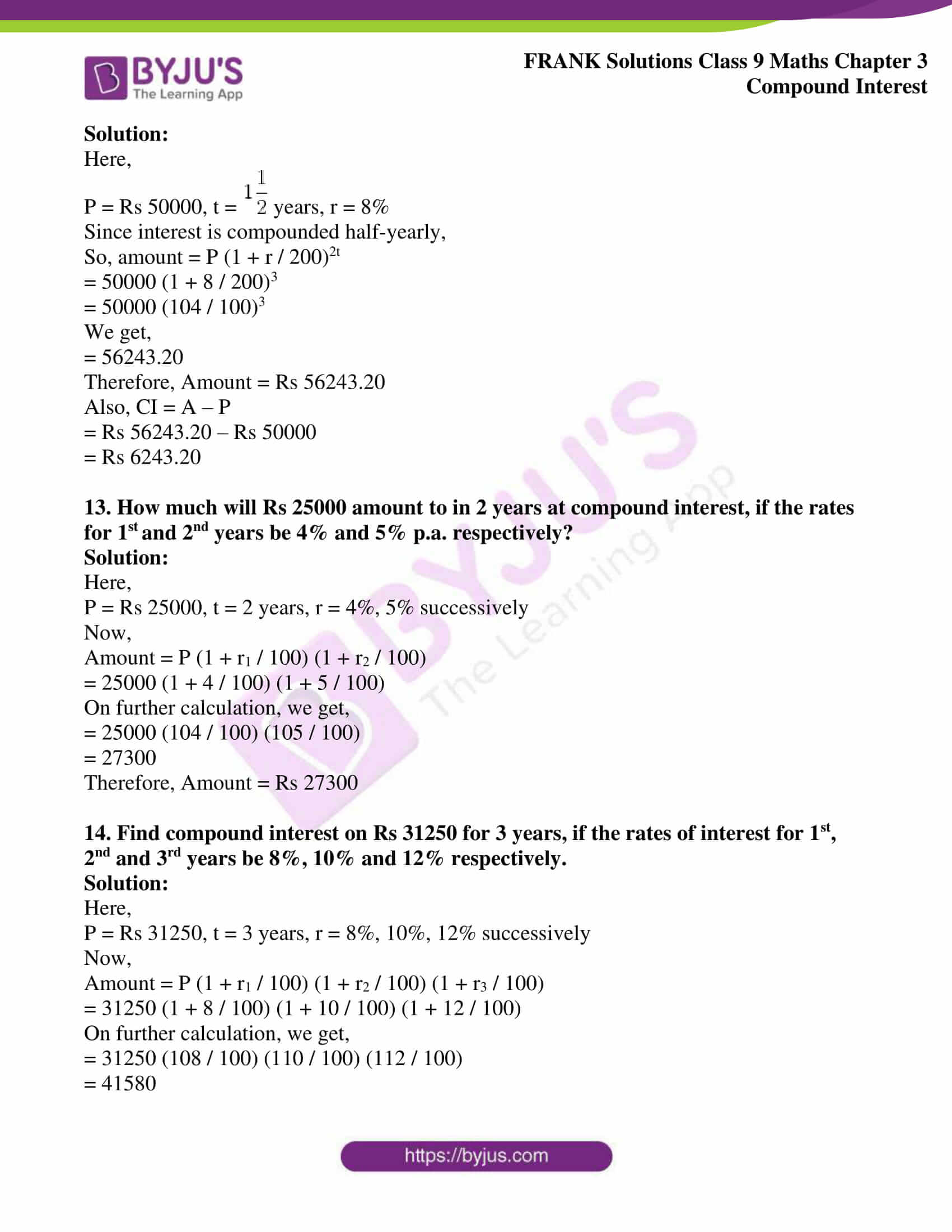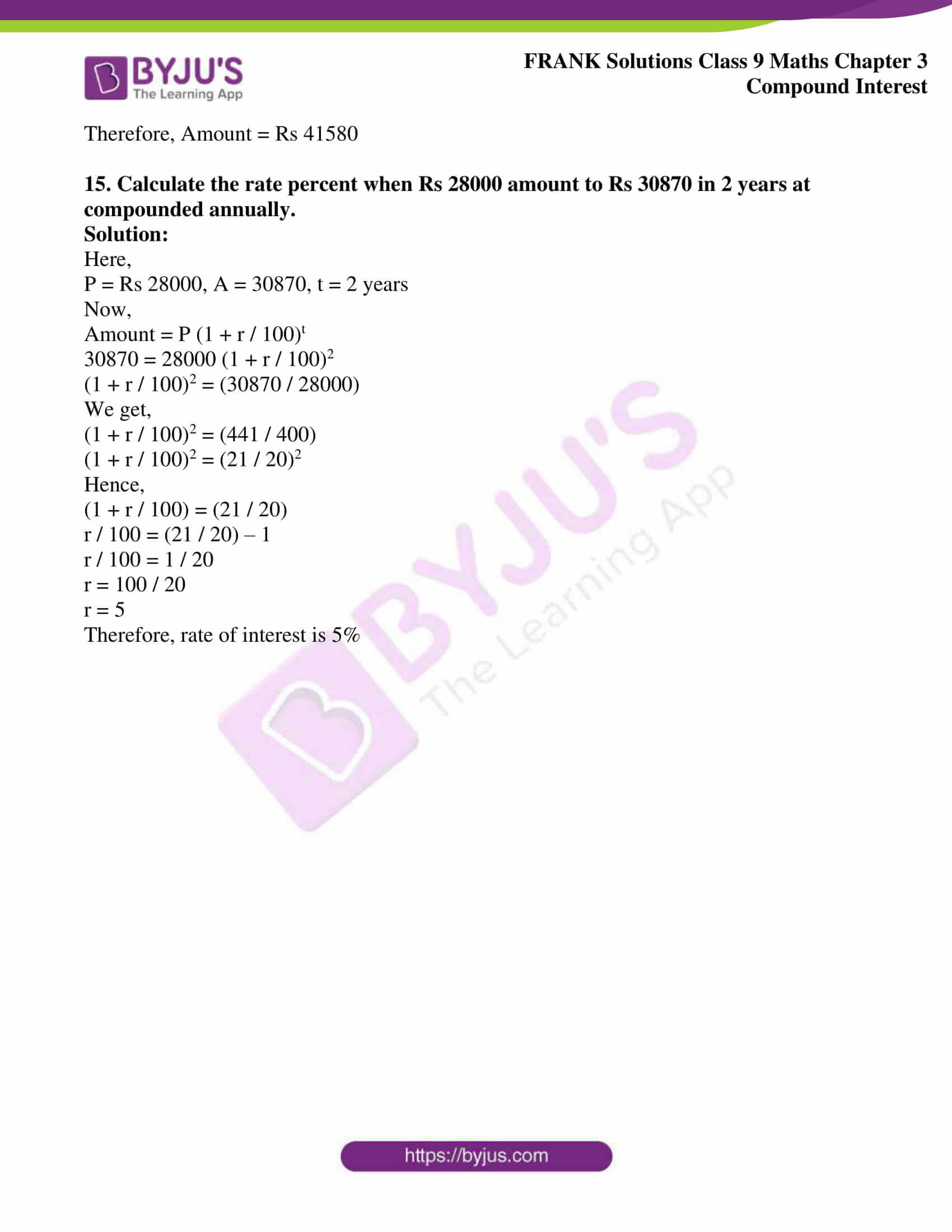## Access Frank Solutions for Class 9 Maths Chapter 3 Compound Interest

1. Find the amount and the compound interest payable annually on the following:

(i) Rs 25000 for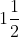years at 10% per annum.

(ii) Rs 32000 for 2 years at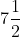% per annum.

(iii) Rs 10000 for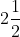years at 6% per annum.

(iv) Rs 24000 foryears at% per annum.

Solution:

(i) Rs 25000 foryears at 10% per annum.

Here,

P = Rs 25000, t =years, r = 10%

Now,

Amount after 1 year = P (1+ r / 100)

= 25000 (1 + 10 / 100)

= 25000 (1 + 1 / 10)

On further calculation, we get,

= 25000 (11 / 10)

= 27500

Hence, the principle for the next 6 months = Rs 27500

Interest for the next 6 months = (27500 × 6 × 10) / (100 × 12)

= 1375

Hence, amount afteryears = Rs 27500 + Rs 1375

= Rs 28875

And CI = A – P

= Rs 28875 – Rs 25000

= Rs 3875

(ii) Rs 32000 for 2 years at% per annum.

Here,

P1 = Rs 32000 and r =% = (15 / 2)%

So, Amount after 1 year = P (1 + r / 100)

= 32000 {1 + 15 / (2 × 100)}

= 32000 (1 + 3 / 40)

= 32000 (43 / 40)

We get,

= 34400

Therefore,

P2 = Rs 34400 and r = (15 / 2)%

So, Amount after 2 year = P (1 + r / 100)

= 34400 {1 + 15 / (2 × 100)}

On further calculation, we get,

= 34400 (1 + 3 / 40)

= 34400 (43 / 40)

We get,

= 36980

Hence, Amount = Rs 36980

And CI = A – P

= Rs 36980 – Rs 32000

We get,

= Rs 4980

(iii) Rs 10000 foryears at 6% per annum.

Here,

P1 = Rs 10000 and r = 6%

So, Amount after 1 year = P (1 + r / 100)

= 10000 (1 + 6 / 100)

= 10000 (106 / 100)

On simplification, we get,

= 10600

Hence, P2 = Rs 10600 and r = 6%

Amount after 2 year = P (1 + r / 100)

= 10600 (1 + 6 / 100)

= 10600 (106 / 100)

On simplification, we get,

= Rs 11236

Hence, the principle for the next 6 months = Rs 11236

Interest for the next 6 months = (11236 × 6 × 6) / (100 × 12)

= 337.08

Hence, the amount afteryears = Rs 11236 + Rs 337.08

= Rs 11573.08

And CI = A – P

= Rs 11573.08 – Rs 10000

= Rs 1573.08

(iv) Rs 24000 foryears at% per annum.

Here,

P = Rs 24000, t =years, r == (15 / 2)%

Now,

Amount after 1 year = P (1 + r / 100)

= 24000 {1 + 15 / (2 × 100)}

= 24000 (1 + 3 / 40)

= 24000 (43 / 40)

We get,

= 25800

Hence, the principle for the next 6 months = Rs 25800

Interest for the next 6 months = (25800 × 15 × 6) / (200 × 12)

= 967.50

Hence, the amount afteryears = Rs 25800 + Rs 967.50

= 26767.50

And CI = A – P

= Rs 26767.50 – Rs 24000

= Rs 2767.50

2. Find the amount and the compound interest payable annually on:

(a) Rs 16000 for 2 years at 15% and 12% for successive years.

(b) Rs 17500 for 3 years at 8%, 10% and 12% for the successive years

Solution:

(a) For first year: P = Rs 16000, R = 15% and T = 1 year

Therefore, interest = Rs (16000 × 15 × 1) / 100

= Rs 2400

And, amount = Rs 16000 + Rs 2400

= Rs 18400

For second year: P = Rs 18400, R = 12% and T = 1 year

Therefore, interest = Rs (18400 × 12 × 1) / 100

= Rs 2208

And, amount = Rs 18400 + Rs 2208

= Rs 20608

Hence, required amount = Rs 20608

And, Compound Interest = A – P

= Rs 20608 – Rs 16000

We get,

= Rs 4608

(b) For first year: P = Rs 17500, R = 8% and T = 1 year

Therefore, interest = Rs (17500 × 8 × 1) / 100

= Rs 1400

And, amount = Rs 17500 + Rs 1400

= Rs 18900

For second year: P = Rs 18900, R = 10% and T = 1 year

Therefore, interest = Rs (18900 × 10 × 1) / 100

= Rs 1890

And, amount = Rs 18900 + Rs 1890

= Rs 20790

For third year: P = Rs 20790, R = 12% and T = 1 year

Therefore, interest = Rs (20790 × 12 × 1) / 100

= Rs 2494.80

And, amount = Rs 20790 + Rs 2494.80

= Rs 23284.80

Hence, required amount = Rs 23284.80

And, Compound Interest = A – P

= Rs 23284.80 – Rs 17500

= Rs 5784.80

3. Calculate the amount and compound interest on Rs 20000 for 3 years at 10% per annum, interest being payable annually.

Solution:

Here,

P1 = Rs 20000 and r = 10%

So, amount after 1 year = P (1 + r / 100)

= 20000 (1 + 10 / 100)

= 20000 (110 / 100)

We get,

= 22000

Hence, P2 = Rs 22000 and r = 10%

Amount after 2 year = P (1 + r / 100)

= 22000 (1 + 10 / 100)

= 22000 (110 / 100)

We get,

= 24200

Hence, P3 = Rs 24200 and r = 10%

Amount after 3 year = P (1 + r / 100)

= 24200 (1 + 10 / 100)

= 24200 (110 / 100)

We get,

= 26620

Therefore, amount = Rs 26620

Also, CI = A – P

= Rs 26620 – Rs 20000

= Rs 6620

4. Compute the compound interest for the third year on Rs 5000 invested for 5 years at 10% per annum, the interest being payable annually.

Solution:

For first year: P = Rs 5000, R = 10% and T = 1 year

Therefore, interest = Rs (5000 × 10 × 1) / 100

= Rs 500

And, amount = Rs 5000 + Rs 500

= Rs 5500

For second year: P = Rs 5500, R = 10% and T = 1 year

Therefore, interest = Rs (5500 × 10 × 1) / 100

= Rs 550

And, amount = Rs 5500 + Rs 550

= Rs 6050

For third year: P = Rs 6050, R = 10% and T = 1 year

Therefore, interest = Rs (6050 × 10 × 1) / 100

= Rs 605

Hence, Compound Interest for the third year is Rs 605

5. Rakesh invests Rs 25600 at 5% per annum compound interest, payable annually for 3 years. Find the amount standing on his credit at the end of the second year.

Solution:

For first year: P = Rs 25600, R = 5% and T = 1 year

Therefore, interest = Rs (25600 × 5 × 1) / 100

= Rs 1280

And, amount = Rs 25600 + Rs 1280

= Rs 26880

For second year: P = Rs 26880, R = 5% and T = 1 year

Therefore, interest = Rs (26880 × 5 × 1) / 100

= Rs 1344

And, amount = Rs 26880 + Rs 1344

= Rs 28224

Therefore, the amount at the end of the second year is Rs 28224

6. Find the amount and compound interest on Rs 7500 foryears at 8%, payable semi-annually.

Solution:

Here,

P1 = Rs 7500 and rate of interest for half year (r) = 4%

So, amount after half year = P (1 + r / 100)

= 7500 (1 + 4 / 100)

= 7500 (104 / 100)

We get,

= 7800

Hence, P2 = Rs 7800 and r = 4%

Amount after 1 year = P (1 + r / 100)

= 7800 (1 + 4 / 100)

= 7800 (104 / 100)

We get,

= 8112

Hence, P3 = Rs 8112 and r = 4%

Amount afteryear = P (1 + r / 100)

= 8112 (1 + 4 / 100)

= 8112 (104 / 100)

We get,

= 8436.48

Therefore, amount = Rs 8436.48

Also, CI = A – P

= Rs 8436.48 – Rs 7500

= Rs 936.48

7. A man invests Rs 24000 for two years at compound interest, if his money amounts to Rs 27600 after one year, find the amount at the end of the second year.

Solution:

Amount after 1 year = P (1 + r / 100)

27600 = 24000 (1 + r / 100)

(1 + r / 100) = 27600 / 24000

We get,

(1 + r / 100) = 23 / 20

On further calculation, we get,

r / 100 = (23 / 20) – 1

We get,

r / 100 = 3 / 20

r = (100 × 3) / 20

r = 15

Amount after 2 year = P (1 + r / 100)

= 27600 {1 + (15 / 100)}

= 27600 (115 / 100)

We get,

= 31740

Therefore, the amount at the end of the second year is Rs 31740

8. How much will Rs 14000 amount to 2 years at compound interest if the rates for the successive years be 5% and 8%, respectively?

Solution:

Here,

P1 = Rs 14000 and r = 5%

So, Amount after 1 year = P (1 + r / 100)

= 14000 (1 + 5 / 100)

= 14000 (105 / 100)

We get,

= 14700

Hence, P2 = Rs 14700 and r = 8%

Amount after 2 year = P (1 + r / 100)

= 14700 (1 + 8 / 100)

= 14700 (108 / 100)

We get,

= 15876

Therefore, amount = Rs 15876

9. Find the amount and the compound interest on the following:

(i) Rs 8000 for 3 years at 10% per annum compounded annually

(ii) Rs 15000 for 2 years at 8% per annum compounded semi-annually

(iii) Rs 12000 foryears at 5% per annum compounded annually

(iv) Rs 25000 for 2 years at 6% per annum compounded semi-annually

(v) Rs 16000 for 3 years at 10%, 8% and 6% for successive years

Solution:

(i) Rs 8000 for 3 years at 10% per annum compounded annually

Here,

P = Rs 8000, t = 3 years, r = 10%

Now,

Amount = P (1 + r / 100)t

= 8000 (1 + 10 / 100)3

= 8000 (11 / 10)3

= 8000 × (1331 / 1000)

We get,

= 10648

Therefore, amount = Rs 10648

Also, CI = A – P

= Rs 10648 – Rs 8000

= Rs 2648

(ii) Rs 15000 for 2 years at 8% per annum compounded semi-annually

Here,

P = Rs 15000, t = 2 years, r = 8%

Since interest is compounded semi-annually, so

Amount = P (1 + r / 200)2t

= 15000 (1 + 8 / 200)4

= 15000 (26 / 25)4

= 15000 × (26 / 25) × (26 / 25) × (26 / 25) × (26 / 25)

On simplification, we get,

= 17547.88

Therefore, amount = Rs 17547.88

Also, CI = A – P

= Rs 17547.88 – Rs 15000

= Rs 2547.88

(iii) Rs 12000 foryears at 5% per annum compounded annually

Here,

P = Rs 12000, t =years, r = 5%

Now,

Amount after 1 year = P (1 + r / 100)t

= 12000 (1 + 5 / 100)

On simplification, we get,

= 12000 (105 / 100)

= 12600

Now, interest for the next half year = (12600 × 5) / (100 × 2)

= 315

Therefore, amount = Rs 12600 + Rs 315

= Rs 12915

Also, CI = A – P

= Rs 12915 – Rs 12000

= Rs 915

(iv) Rs 25000 for 2 years at 6% per annum compounded semi-annually

Here,

P = Rs 25000, t = 2 years, r = 6%

Since interest is compounded semi-annually,

Amount = P (1 + r / 200)2t

= 25000 (1 + 6 / 200)4

= 25000 (103 / 100)4

On simplification, we get,

= 28137.72

Hence, amount = Rs 28137.72

Also, CI = A – P

= Rs 28137.72 – Rs 25000

= Rs 3137.72

(v) Rs 16000 for 3 years at 10%, 8% and 6% for successive years

Here,

P = Rs 16000, t = 3 years, r = 10%, 8%, 6% successively

Now,

Amount = P (1 + r1 / 100) (1 + r2 / 100) (1 + r3 / 100)

= 16000 (1 + 10 / 100) (1 + 8 / 100) (1 + 6 / 100)

On simplification, we get,

= 16000 (11 / 10) (108 / 100) (106 / 100)

= 20148.48

Therefore, Amount = Rs 20148.48

Also, CI = A – P

= Rs 20148.48 – Rs 16000

= Rs 4148.48

10. Find the amount and compound interest on Rs 15000 inyears at 10% p.a. compounded annually

Solution:

Here,

P = Rs 15000, t =years, r = 10%

Now, Amount after 2 year = P (1 + r / 100)t

= 15000 (1 + 10 / 100)2

= 15000 (11 / 10)2

We get,

= 18150

Now, interest for the next half year = (18150 × 10) / (100 × 2)

= 907.5

Therefore, Amount = Rs 18150 + Rs 907.50

= Rs 19057.50

Also, CI = A – P

= Rs 19057.50 – Rs 15000

= Rs 4057.50

11. Find the amount of Rs 36000 in 2 years 15% p.a. compounded annually.

Solution:

Here,

P = Rs 36000, t = 2 years, r = 15%

Now,

Amount = P (1 + r / 100)t

= 36000 (1 + 15 / 100)2

= 36000 (115 / 100)2

We get,

= 47610

Therefore, amount = Rs 47610

12. Find the amount and compound interest on Rs 50000 inyears at 8% p.a. compounded half-yearly.

Solution:

Here,

P = Rs 50000, t =years, r = 8%

Since interest is compounded half-yearly,

So, amount = P (1 + r / 200)2t

= 50000 (1 + 8 / 200)3

= 50000 (104 / 100)3

We get,

= 56243.20

Therefore, Amount = Rs 56243.20

Also, CI = A – P

= Rs 56243.20 – Rs 50000

= Rs 6243.20

13. How much will Rs 25000 amount to in 2 years at compound interest, if the rates for 1st and 2nd years be 4% and 5% p.a., respectively?

Solution:

Here,

P = Rs 25000, t = 2 years, r = 4%, 5% successively

Now,

Amount = P (1 + r1 / 100) (1 + r2 / 100)

= 25000 (1 + 4 / 100) (1 + 5 / 100)

On further calculation, we get,

= 25000 (104 / 100) (105 / 100)

= 27300

Therefore, Amount = Rs 27300

14. Find the compound interest on Rs 31250 for 3 years, if the rates of interest for 1st, 2nd and 3rd years be 8%, 10% and 12%, respectively.

Solution:

Here,

P = Rs 31250, t = 3 years, r = 8%, 10%, 12% successively

Now,

Amount = P (1 + r1 / 100) (1 + r2 / 100) (1 + r3 / 100)

= 31250 (1 + 8 / 100) (1 + 10 / 100) (1 + 12 / 100)

On further calculation, we get,

= 31250 (108 / 100) (110 / 100) (112 / 100)

= 41580

Therefore, Amount = Rs 41580

15. Calculate the rate per cent when Rs 28000 amounts to Rs 30870 in 2 years at compounded annually.

Solution:

Here,

P = Rs 28000, A = 30870, t = 2 years

Now,

Amount = P (1 + r / 100)t

30870 = 28000 (1 + r / 100)2

(1 + r / 100)2 = (30870 / 28000)

We get,

(1 + r / 100)2 = (441 / 400)

(1 + r / 100)2 = (21 / 20)2

Hence,

(1 + r / 100) = (21 / 20)

r / 100 = (21 / 20) – 1

r / 100 = 1 / 20

r = 100 / 20

r = 5

Therefore, the rate of interest is 5%